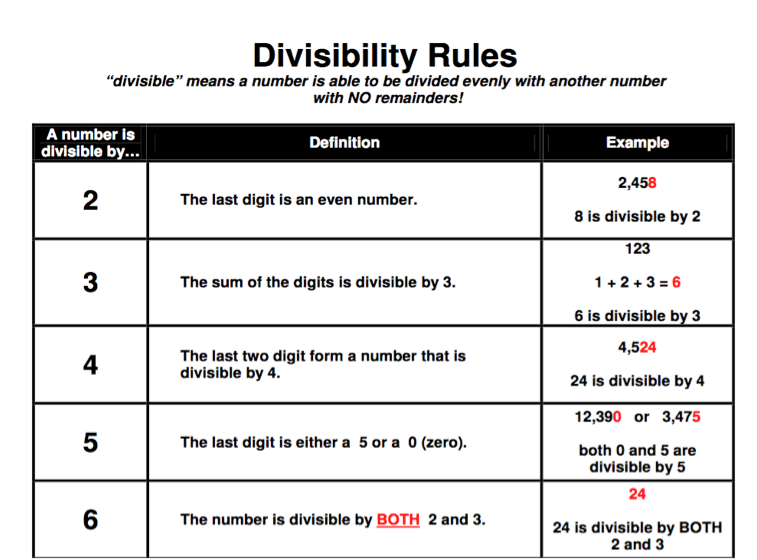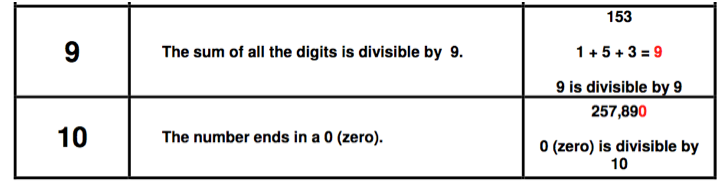## Homework

•  Math May
• ratios and proportions
• Math  May 14
B/C Class  Complete Weekly home work  Tue.
F/G Class  Complete Weekly home work  Tue.

• Weekly homework to be passed in on Friday

Science
A period    Complete Question page 446

• # This month's highlights

Write word sentences as equations.

Use substitution to check answers.

Identify independent and dependent variables.

Write equations in two variables.

Use tables and graphs to analyze the relationship between two variables.

Write word sentences as inequalities.

Use a number line to graph the solution set of inequalities.

Use inequalities to represent real-life situations.

Use addition or subtraction to solve inequalities.

Use a number line to graph the solution set of inequalities.

Use multiplication or division to solve inequalities.

• # Videos

• https://youtu.be/6fYn6qV-GT8

• https://youtu.be/hwU3YL_SD70

• https://youtu.be/x0E4vxLydNY

• https://youtu.be/LcS9dQezx4A

https://www.helpingwithmath.com/by_subject/division/div_divisibility_rules.htm• 3-18
• B/C Class  Practice B 6.1
• F/G Class  no homework
• 3-18
• B/C Class  Practice B 6.1
• F/G Class  no homework
• 3-18
• B/C Class  Practice B 6.1
• F/G Class  no homework